# Simplify 2

Simplify expression: 5ab-7+3ba-9

Result

e = (Correct answer is: 8ab-16)### Step-by-step explanation: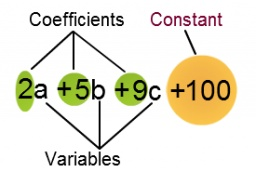Did you find an error or inaccuracy? Feel free to write us. Thank you!

Showing 1 comment:Lj Grim
this website is really fun and gets you ahead of math and you can even use this for some answers or examples that you need to know## Related math problems and questions:

• Simplify:Simplify: 8(−9 − 5x) + 4b use x = 9 and b = −9
• SimplifySimplify expression - which expression is equivalent to: 3(m + 2) − 4(2m − 9)
• SimplifySimplify the following problem and express as a decimal: 5.68-[5-(2.69+5.65-3.89) /0.5]
• What is bigger?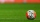Which ball has a larger volume: a football with a circumference of 66 cm or a volleyball with a diameter of 20 cm?
• Interst on savings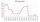The bank offers 1.6% interest. How many euros have to insert at the beginning if we received € 15 on the interest?
• Truncated coneCalculate the height of the rotating truncated cone with volume V = 794 cm3 and a base radii r1 = 9.9 cm and r2 = 9.8 cm.
• Expressions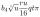Expression (3a-7).(3a+7) is identical with: ?After the history test, Michaella discovered that the ratio of her correct and incorrect answers is 5: 3. How many correct answers did Michaella have in the test, if she had 6 incorrect answers?
• Medians 2:1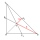Median to side b (tb) in triangle ABC is 12 cm long. a. What is the distance of the center of gravity T from the vertex B? b, Find the distance between T and the side b.
• The depositDeposit has been placed for one year at an annual rate of 4.5%. After the added interst amount rose to € 2,612.5. Determine initial deposit.
• Value of expressionX=2, y=-5 and z=3 what is the value of x-2y?
• WhichWhich decimals when subtracted equal 3.89: a - b = 3.89
• Simplify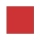Simplify powers multiplication: (3+22)(5-42)
• Simplify 7Simplify. 7-1/3÷ 3-2/3 of 2+ 4-1/2÷ 2-1/4+ 1/2 solution and by step by step
• Math testIn mathematics, there were 25 problems of three kinds: light 2 points, medium 3 points, heavy 5 points, the best score is 84 points. How many points did Jane have when she solved all the easy examples, half medium and one-third difficult?
• Unknown number 17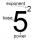Milada said, I am thinking of a number such that I evaluate expression x1/3, the value of the expression would be 5. Which number Milada thinking?
• The quotient 2The quotient of the quantity k minus 10 and m